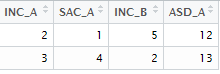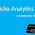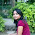# R : Keep / Drop Columns from Data Frame

The article below explains how to keep or drop variables (columns) from data frame. In R, there are multiple ways to select or drop column.

Create a sample data frame

The following code creates a sample data frame that is used for demonstration.
set.seed(456)
mydata <- data.frame(a=letters[1:5], x=runif(5,10,50), y=sample(5), z=rnorm(5))Sample Data

R : Delete column by name

Method I :

The most easiest way to drop columns is by using subset() function. In the code below, we are telling R to drop variables x and z. The '-' sign indicates dropping variables. Make sure the variable names would NOT be specified in quotes when using subset() function.
df = subset(mydata, select = -c(x,z) )
```  a y
1 a 2
2 b 1
3 c 4
4 d 3
5 e 5```

Method II :

In this method, we are creating a character vector named drop in which we are storing column names x and z. Later we are telling R to select all the variables except the column names specified in the vector drop. The function names() returns all the column names and the '!' sign indicates negation.
drop <- c("x","z")
df = mydata[,!(names(mydata) %in% drop)]
It can also be written like :  df = mydata[,!(names(mydata) %in% c("x","z"))]

R : Drop columns by column index numbers

It's easier to remove variables by their position number. All you just need to do is to mention the column index number. In the following code, we are telling R to drop variables that are positioned at first column, third and fourth columns. The minus sign is to drop variables.
df <- mydata[ -c(1,3:4) ]
```         x
1 13.58206
2 18.42049
3 39.31821
4 44.08534
5 41.53592
```

R : Keep column by name

Method I :

In this section, we are retaining variables x and z.
keeps <- c("x","z")
df = mydata[keeps]
The above code is equivalent to df = mydata[c("x","z")]

Method II :

We can keep variables with subset() function.
df = subset(mydata, select = c(x,z))

Keep columns by column index number

In this case, we are telling R to keep only variables that are placed at second and fourth position.
df <- mydata[c(2,4)]

Keep or Delete columns with dplyr package

In R, the dplyr package is one of the most popular package for data manipulation. It makes data wrangling easy. You can install package by using the command below -
install.packages("dplyr")

1. How to delete first, third and fourth column
library(dplyr)
mydata2 = select(mydata, -1, -3:-4)
2. How to delete columns a, x and y

This can be written in three ways -
mydata2 = select(mydata, -a, -x, -y)
mydata2 = select(mydata, -c(a, x, y))
mydata2 = select(mydata, -a:-y)

3. How to keep columns a, y and z
mydata2 = select(mydata, a, y:z)

Keep / Drop Columns by Name Pattern

The code below creates data for 4 variables named as follows :
INC_A   SAC_A   INC_B   ASD_A
INC_A SAC_A INC_B ASD_A
2 1 5 12
3 4 2 13Keep / Drop Columns by pattern

Keeping columns whose name starts with "INC"
mydata1 = mydata[,grepl("^INC",names(mydata))]
The grepl() function is used to search for matches to a pattern. In this case, it is searching "INC" at starting in the column names of data frame mydata. It returns INC_A and INC_B.

Dropping columns whose name starts with "INC"

mydata2 = mydata[,!grepl("^INC",names(mydata))]

Keeping columns whose name contain "_A" at the end

The "\$" is used to search for the sub-strings at the end of string. It returns INC_A, SAC_A and ASD_A.
mydata12 = mydata[,grepl("_A\$",names(mydata))]

Dropping columns whose name contain "_A" at the end
mydata22 = mydata[,!grepl("_A\$",names(mydata))]

Keeping columns whose name contain the letter "S"
mydata32 = mydata[,grepl("*S",names(mydata))]
The same logic can be applied to a word as well if you wish to find out columns containing a particular word. In the example below, we are trying to keep columns where it contains `C_A` and creates a new dataframe for the retained columns.
```mydata320 = mydata[,grepl("*C_A",names(mydata))]
```

Dropping columns whose name contain the letter "S"
mydata33 = mydata[,!grepl("*S",names(mydata))]

Drop columns having more than 50% missing values
I have created a dummy data frame which includes several missing or blank values for illustration.
```df= data.frame(x=c(1,2,3,NA,NA), y=c(5,NA,3,NA,NA), Z=c(5,3,3,4,NA))
```
```  x  y  Z
1  1  5  5
2  2 NA  3
3  3  3  3
4 NA NA  4
5 NA NA NA
```
`sapply` function is an alternative of `for loop`. It runs a built-in or user-defined function on each column of data frame. `sapply(df, function(x) mean(is.na(x)))` returns percentage of missing values in each column in your dataframe.
```df = df[,!sapply(df, function(x) mean(is.na(x)))>0.5]
```
The above program removed column Y as it contains 60% missing values more than our threshold of 50%. Output is given below.
```  x  Z
1  1  5
2  2  3
3  3  3
4 NA  4
5 NA NA
```
R Function : Keep / Drop Column Function

The following program automates keeping or dropping columns from a data frame.
```KeepDrop = function(data=df,cols="var",newdata=df2,drop=1) {

# Double Quote Output Dataset Name
t = deparse(substitute(newdata))

# Drop Columns
if(drop == 1){
newdata = data [ , !(names(data) %in% scan(textConnection(cols), what="", sep=" "))]}

# Keep Columns
else {
newdata = data [ , names(data) %in% scan(textConnection(cols), what="", sep=" ")]}
assign(t, newdata, .GlobalEnv)

}
```

How to use the above function

To keep variables 'a' and 'x', use the code below. The drop = 0 implies keeping variables that are specified in the parameter "cols". The parameter "data" refers to input data frame. "cols" refer to the variables you want to keep / remove. "newdata" refers to the output data frame.
KeepDrop(data=mydata,cols="a x", newdata=dt, drop=0)

To drop variables, use the code below. The drop = 1 implies removing variables which are defined in the second parameter of the function.
KeepDrop(data=mydata,cols="a x", newdata=dt, drop=1)Related PostsDeepanshu founded ListenData with a simple objective - Make analytics easy to understand and follow. He has over 8 years of experience in data science. During his tenure, he has worked with global clients in various domains like Banking, Insurance, Telecom and Human Resource.

16 Responses to "R : Keep / Drop Columns from Data Frame"
1.df =df[,-c(21,6,5,7,15,2,3)]

this can work too
these no. are the column number

2.Great summary, thank you!

1.Thank you for stopping by my blog!

3.Best computer science site ever, Thank you from Quito Ecuador.

1.4.Good examples !!!!

1.5.It was nice and easy to understand

6.How to remove a columns which have more than 75% missing values using which()

1.I have added desired details in article. Thanks!

7.If I want to remove all the cells containing "other" From a given data frame. How to create this dataset??

8.I am trying to remove columns where all variables are below a threshold of 5. Any advice?

1.What do you mean by "variables are below a threshold of 5"? Is it based on the number of rows in a variable?

9.Very well explained. Now I am able to do data preprocessing on my own :-). Thank You for sharing.

10.Hi I want to ask something, I want to delete all the values ​​in a column from all the data I do not want. More precisely, I will delete all values ​​of that column in the data, how can I do that?

1.Loans\$LoanID <- NA

Next → ← Prev
Love this Post? Spread the Word!
Share